# PSEB 6th Class Maths Solutions Chapter 10 Practical Geometry Ex 10.6

Punjab State Board PSEB 6th Class Maths Book Solutions Chapter 10 Practical Geometry Ex 10.6 Textbook Exercise Questions and Answers.

## PSEB Solutions for Class 6 Maths Chapter 10 Practical Geometry Ex 10.6

1. Draw a line XY and point P not lying on XY. Draw a line parallel to XY passing through P with the help of ruler and compasses.
Solution:
Steps of Construction:
1. Draw a line XY and point P not lying on it.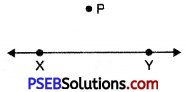2. Take any point Q, anywhere on line XY.
3. Join PQ.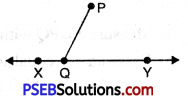4. Now take Q as centre, draw arc AB of any radius on XY. Similarly, draw an arc CD of same radius on line segment PQ from point P.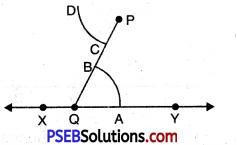5. Measure arc AB with compasses.
6. Draw an arc equal to radius AB from point C witch intersect CD on E.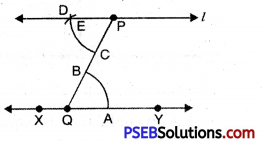7. Join PE and produce it. So, the line l is the required line parallel to XY.2. Draw a line p parallel to line m passing through a point A which is not lying on line m with the help of set squares.
Solution:
Steps of Construction:
1. Given a line m with point A not lying on it.
2. Place one of the edge of a ruler along the line m and hold it firmly.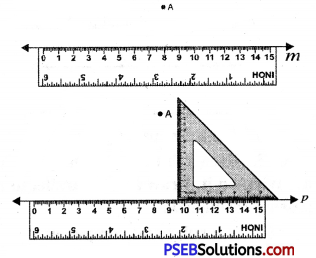3. Place the set square in such a way that one of its edges containing the right angle coincides with the ruler.
4. Hold the ruler firmly, slide the set square along the line m till its vertical side reaches the point A.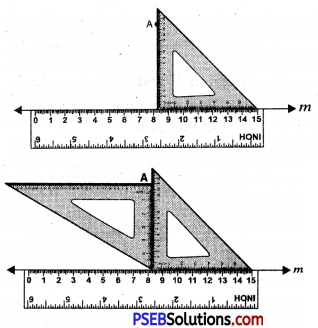5. Firmly hold the set square in this position, take another set square and place it in such a way that one of its edges containing right angle concides with previous set square as shown.
6. Now draw a line p along side of second set square passing through A.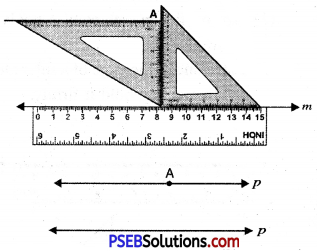Thus p $$\text { ॥ }$$ m passing through A.3. Given a line AB and the point X is not lying on it. Draw a line parallel to AB passing through X.

Question (i)
By a ruler and compasses
Solution:
By a ruler and compasses:
Let us consider a line AB and point X not lying on it.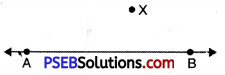Steps of Construction:
1. Take any point, say Y anywhere on line AB.
2. Join XY.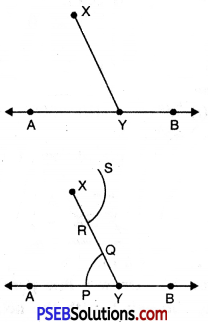3. Now take Y as centre, draw an arc PQ of any radius on AB. Similarly draw an arc RS of same radius on line segment XY from point X.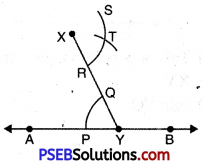4. Measure arc PQ with compasses.
5. Draw an arc equal to radius PQ from point R which intersect RS on T.
6. Join XT and produce it.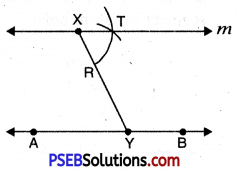So the line m is the required line parallel to AB.Question (ii)
By set squares.
Solution:
By set squares.

Steps of Construction:
1. Given a line AB with point X not lying on it.
2. Place one of the edge of a ruler along the line AB and hold it firmly.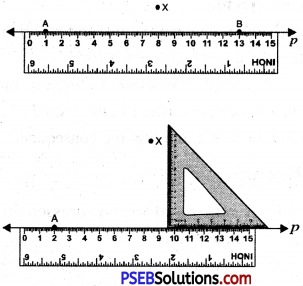3. Place the set square in such a way that one of its edges containing the right angle coincides with the ruler.
4. Hold the ruler firmly, slide the set square along the line AB till its vertical side reaches the point X.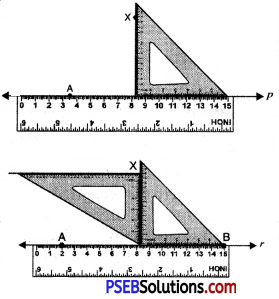5. Firmly hold the set square in this position, take another set square and place it in such a way that one of its edges containing right angle concides with previous set square as shown.
6. Now draw a line l along side of second set square passing through X.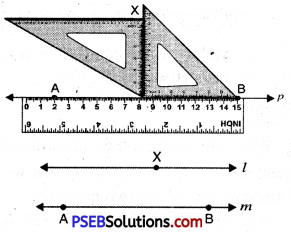7. Thus l $$\text { ॥ }$$ AB passing through X.